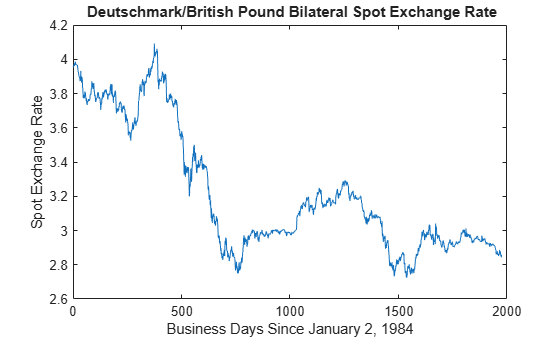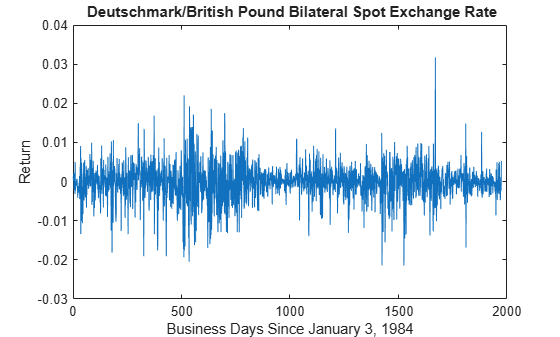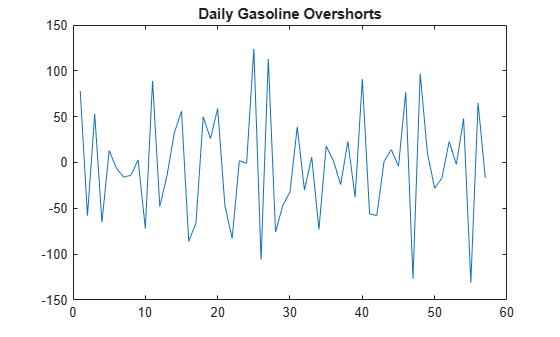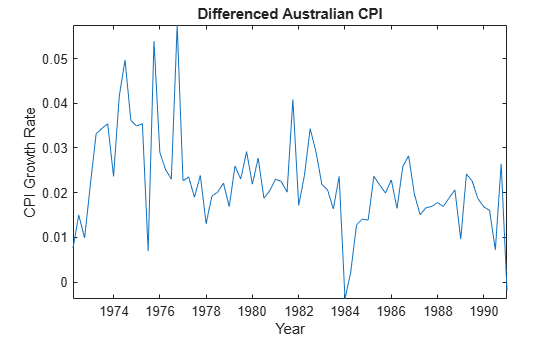# lbqtest

Ljung-Box Q-test for residual autocorrelation

## Syntax

h = lbqtest(res)
[h,pValue,stat,cValue] = lbqtest(res)
StatTbl = lbqtest(Tbl)
[___] = lbqtest(___,Name=Value)

## Description

example

h = lbqtest(res) returns the rejection decision h from conducting a Ljung-Box Q-test for autocorrelation in the residual series res.

example

[h,pValue,stat,cValue] = lbqtest(res) also returns the p-value pValue, test statistic stat, and critical value cValue of the test.

example

StatTbl = lbqtest(Tbl) returns the table StatTbl containing variables for the test results, statistics, and settings from conducting a Ljung-Box Q-test for residual autocorrelation in the last variable of the input table or timetable Tbl. To select a different variable in Tbl to test, use the DataVariable name-value argument.

example

[___] = lbqtest(___,Name=Value) specifies options using one or more name-value arguments in addition to any of the input argument combinations in previous syntaxes. lbqtest returns the output argument combination for the corresponding input arguments.Some options control the number of tests to conduct. The following conditions apply when lbqtest conducts multiple tests: lbqtest treats each test as separate from all other tests.If you specify res, all outputs are vectors.If you specify Tbl, each row of StatTbl contains the results of the corresponding test. For example, lbqtest(Tbl,DataVariable="ResidualGDP",Alpha=0.025,Lags=[1 4]) conducts two tests, at a level of significance of 0.025, for the presence of residual autocorrelation in the variable ResidualGDP of the table Tbl. The first test includes 1 lag in the test statistic, and the second test includes 4 lags.

## Examples

collapse all

Test a time series for residual autocorrelation using default options of lbqtest. Input the time series data as a numeric vector.

Load the Deutschmark/British pound foreign-exchange rate data set.

load Data_MarkPound

Data is a time series vector of daily Deutschmark/British pound bilateral spot exchange rates.

Plot the series.

plot(Data) title("\bf Deutschmark/British Pound Bilateral Spot Exchange Rate") ylabel("Spot Exchange Rate") xlabel("Business Days Since January 2, 1984")The series appears nonstationary.

To stabilize the series, convert the spot exchange rates to returns.

returns = price2ret(Data); plot(returns) title("\bf Deutschmark/British Pound Bilateral Spot Exchange Rate") ylabel("Return") xlabel("Business Days Since January 3, 1984")Compute the deviations of the return series from the mean.

residuals = returns - mean(returns);

At 0.05 level of significance, test the residual series for autocorrelation using the default options of the Ljung-Box Q-test.

h = lbqtest(residuals)
h = logical 0 

The result h = 0 indicates that insufficient evidence exists to reject the null hypothesis of no residual autocorrelation through 20 lags.

Load the Deutschmark/British pound foreign-exchange rate data set.

load Data_MarkPound

Preprocess the data by following this procedure:

1. Stabilize the series by computing daily returns.

2. Compute the deviations from the mean return.

returns = price2ret(Data); residuals = returns - mean(returns);

Test the residual series for a significant autocorrelation from 1 through 20 lags. Return the test decision, $\mathit{p}$-value, test statistic, and critical value.

[h,pValue,stat,cValue] = lbqtest(residuals)
h = logical 0 
pValue = 0.1131 
stat = 27.8445 
cValue = 31.4104 

Test a time series, which is one variable in a table, for residual autocorrelation using default options of lbqtest.

Load the equity index data set Data_EquityIdx. Preprocess the daily NASDAQ closing prices by performing the following actions:

1. Convert the price series to a percentage return series by using price2ret.

2. Represent the series as residuals that fluctuate around a constant level by centering the returns series.

Store the residual series in the table with the rest of the data. Because the price-to-return conversion reduces the sample size from the head of the series, replace the missing residual with the a NaN.

load Data_EquityIdx ret = 100*price2ret(DataTable.NASDAQ); res = ret - mean(ret); DataTable.Residuals_NASDAQ = [NaN; res]; DataTable.Properties.VariableNames{end}
ans = 'Residuals_NASDAQ' 

The residual series is the last variable in the table.

Conduct Ljung-Box Q-test on the residual series at a 5% significance level by supplying the entire data set lbqtest.

StatTbl = lbqtest(DataTable)
StatTbl=1×7 table h pValue stat cValue Lags Alpha DoF _____ __________ ______ ______ ____ _____ ___ Test 1 true 2.8182e-11 92.395 31.41 20 0.05 20 

lbqtest returns test results and settings in the table StatTbl, where variables correspond to test results (h, pValue, stat, and cValue) and settings (Lags, Alpha, and DoF), and rows correspond to individual tests (in this case, lbqtest conducts one test).

h = 1 and pValue = 2.82e-11 rejects the null hypothesis and suggests that the evidence for at least one significant autocorrelation in lags 1 through 20 in the NASDAQ returns residual series is strong.

By default, lbqtest tests the last variable in the table. To select a variable from an input table to test, set the DataVariable option.

Load the Deutschmark/British pound foreign-exchange rate data set.

load Data_MarkPound

Convert the prices to returns.

returns = price2ret(Data);

Compute the deviations of the return series.

res = returns - mean(returns);

Test the hypothesis that the residual series is not autocorrelated, using the default number of lags.

h1 = lbqtest(res)
h1 = logical 0 

h1 = 0 indicates that there is insufficient evidence to reject the null hypothesis that the residuals of the returns are not autocorrelated.

Test the hypothesis that there are significant ARCH effects, using the default number of lags .

h2 = lbqtest(res.^2)
h2 = logical 1 

h2 = 1 indicates that there are significant ARCH effects in the residuals of the returns.

Test for residual heteroscedasticity using archtest. Specify an alternative ARCH($\mathit{L}$) model, where $\mathit{L}\le \text{\hspace{0.17em}}\mathrm{20}$, for consistency with h2.

h3 = archtest(res,Lags=20)
h3 = logical 1 

h3 = 1 indicates that the null hypothesis of no residual heteroscedasticity should be rejected in favor of an ARCH($\mathit{L}$) model, where $\mathit{L}\le \text{\hspace{0.17em}}\mathrm{20}$. This result is consistent with h2.

Conduct multiple Ljung-Box Q-tests for autocorrelation by specifying several lags for the test statistic. The data set is a time series of 57 consecutive days of overshorts from an underground gasoline tank in Colorado . That is, the current overshort (${y}_{t}$) represents the accuracy in measuring the amount of fuel:

• In the tank at the end of day $t$

• In the tank at the end of day $t-1$

• Delivered to the tank on day $t$

• Sold on day $t$.

load Data_Overshort T = height(DataTable); figure plot(DataTable.OSHORT) title('Daily Gasoline Overshorts')lbqtest is appropriate for a series with a constant mean. Because the series appears to fluctuate around a constant mean, you do not need to stabilize it.

Compute deviations from the mean.

DataTable.Residuals_OSHORT = DataTable.OSHORT - mean(DataTable.OSHORT);

Assess whether the residuals are autocorrelated. Include 5, 10, and 15 lags in the test statistic, and adjust the significance level of each test to 0.05/3.

StatTbl = lbqtest(DataTable,DataVariable="Residuals_OSHORT", ... Lags=[5 10 15],Alpha=0.05/3)
StatTbl=3×7 table h pValue stat cValue Lags Alpha DoF _____ __________ ______ ______ ____ ________ ___ Test 1 true 0.0016465 19.36 13.839 5 0.016667 5 Test 2 true 0.00068328 30.599 21.707 10 0.016667 10 Test 3 true 0.001281 36.964 28.88 15 0.016667 15 

Rows of StatTbl contain results of separate tests conducted for each specified lag. Each test rejects the null hypothesis at 0.0167 level of significance.

Infer residuals from an estimated ARIMA model, and assess whether the residuals exhibit autocorrelation using lbqtest.

Load the Australian Consumer Price Index (CPI) data set. The time series (cpi) is the log quarterly CPI from 1972 to 1991. Remove the trend in the series by taking the first difference.

load Data_JAustralian cpi = DataTable.PAU; T = length(cpi); dCPI = diff(cpi); dt = datetime(dates,ConvertFrom="datenum"); figure plot(dt(2:T),dCPI) title("Differenced Australian CPI") xlabel("Year") ylabel("CPI Growth Rate") axis tightThe differenced series appears stationary.

Fit an AR(1) model to the series, and then infer residuals from the estimated model.

Mdl = arima(1,0,0); EstMdl = estimate(Mdl,dCPI);
 ARIMA(1,0,0) Model (Gaussian Distribution): Value StandardError TStatistic PValue _________ _____________ __________ __________ Constant 0.015564 0.0028766 5.4106 6.2808e-08 AR{1} 0.29646 0.11048 2.6834 0.0072876 Variance 0.0001038 1.1932e-05 8.6994 3.3362e-18 
res = infer(EstMdl,dCPI); stdRes = res/sqrt(EstMdl.Variance); % Standardized residuals

Assess whether the residuals are autocorrelated by conducting a Ljung-Box Q-test. The standardized residuals originate from the estimated model (EstMdl) containing parameters. When using such residuals, perform the following actions:

• Adjust the degrees of freedom (DoF) of the test statistic distribution to account for the estimated parameters.

• Set the number of lags to include in the test statistic.

• When you count the estimated parameters, skip the constant and variance parameters.

lags = 10; dof = lags - 1; % One autoregressive parameter [h,pValue] = lbqtest(stdRes,Lags=lags,DoF=dof)
h = logical 1 
pValue = 0.0119 

pValue = 0.0119 suggests that the residuals have significant autocorrelation in at least one lag of lags 1 through 5, at the 5% level.

## Input Arguments

collapse all

Residual series, specified as a numeric vector. Each element of res corresponds to an observation.

Typically, res contains the (standardized) residuals from a model fit to observed time series.

Data Types: double

Time series data, specified as a table or timetable. Each row of Tbl is an observation.

Specify a single residual series (variable) to test by using the DataVariable argument. The selected variable must be numeric.

Note

Specify missing observations using NaN. The lbqtest function treats missing values as missing completely at random.

### Name-Value Arguments

Specify optional pairs of arguments as Name1=Value1,...,NameN=ValueN, where Name is the argument name and Value is the corresponding value. Name-value arguments must appear after other arguments, but the order of the pairs does not matter.

Before R2021a, use commas to separate each name and value, and enclose Name in quotes.

Example: lbqtest(Tbl,DataVariable="ResidualGDP",Alpha=0.025,Lags=[1 4]) conducts two tests, at a level of significance of 0.025, for the presence of residual autocorrelation in the variable ResidualGDP of the table Tbl. The first test includes 1 lag in the test statistic, and the second test includes 4 lags.

Number of lags L to include in the test statistic, specified as a positive integer that is less than T or a vector of such positive integers, where T is the effective sample size (the number of nonmissing values in the input series).

lbqtest conducts a separate test for each element in Lags.

Example: Lags=[1 4] conducts two tests. The first test includes only the first lag in the AR model of the squared residuals, and the second test includes the first through fourth lags.

Data Types: double

Significance level for the hypothesis test, specified as a numeric scalar in the interval (0,1) or a numeric vector of such values.

lbqtest conducts a separate test for each value in Alpha.

Example: Alpha=[0.01 0.05] uses a level of significance of 0.01 for the first test, and then uses a level of significance of 0.05 for the second test.

Data Types: double

Degrees of freedom for the asymptotic chi-square distribution of the test statistic under the null hypothesis, specified as a positive integer or vector of positive integers.

lbqtest conducts a separate test for each value in DoF.

If DoF is an integer, then it must be less than or equal to Lags. Otherwise, each element of DoF must be less than or equal to the corresponding element of Lags.

Example: DoF=15 specifies 15 degrees of freedom for the distribution of the test statistic.

Data Types: double

Variable in Tbl to test, specified a string scalar or character vector containing a variable name in Tbl.Properties.VariableNames, or an integer or logical vector representing the index of a name.

Example: DataVariable="ResidualGDP"

Example: DataVariable=[false true false false] or DataVariable=2 tests the second table variable.

Data Types: double | logical | char | string

## Output Arguments

collapse all

Test rejection decisions, returned as a logical scalar or vector with length equal to the number of tests. lbqtest returns h when you supply the input res.

• Values of 1 indicate rejection of the no residual autocorrelation null hypothesis in favor of the alternative.

• Values of 0 indicate failure to reject the no residual autocorrelation null hypothesis.

Test statistic p-values, returned as a numeric scalar or vector with length equal to the number of tests. lbqtest returns pValue when you supply the input res.

Test statistics, returned as a numeric scalar or vector with length equal to the number of tests. lbqtest returns stat when you supply the input res.

Test critical values, determined by Alpha, returned as a numeric scalar or vector with length equal to the number of tests. lbqtest returns cValue when you supply the input res.

Test summary, returned as a table with variables for the outputs h, pValue, stat, and cValue, and with a row for each test. lbqtest returns StatTbl when you supply the input Tbl.

StatTbl contains variables for the test settings specified by Lags, Alpha, and DoF.

collapse all

### Ljung-Box Q-Test

The Ljung-Box Q-test is a "portmanteau" test that assesses the null hypothesis that a series of residuals exhibits no autocorrelation for a fixed number of lags L (see Lags), against the alternative that some autocorrelation coefficient ρ(k), k = 1, ..., L is nonzero.

The test statistic is

$Q=T\left(T+2\right)\sum _{k=1}^{L}\left(\frac{\rho {\left(k\right)}^{2}}{\left(T-k\right)}\right),$

where T is the sample size, L is the number of autocorrelation lags, and ρ(k) is the sample autocorrelation at lag k. Under the null hypothesis, the asymptotic distribution of Q is chi-square with L degrees of freedom.

### Missing Completely at Random

Observations of a random variable are missing completely at random if the tendency of an observation to be missing is independent of both the random variable and the tendency of all other observations to be missing.

## Tips

If you obtain the input residual series by fitting a model to data, reduce the degrees of freedom DoF by the number of estimated coefficients, excluding constants. For example, if you obtain the input residuals by fitting an ARMA(p,q) model, set DoF=Lpq, where L is the value of Lags.

## Algorithms

• The value of the Lags argument L affects the power of the test.

• If L is too small, the test does not detect high-order autocorrelations.

• If L is too large, the test loses power when a significant correlation at one lag is washed out by insignificant correlations at other lags.

• Box, Jenkins, and Reinsel suggest the default setting Lags=min[20,T-1] .

• Tsay cites simulation evidence showing better test power performance when L is approximately log(T) .

• lbqtest does not directly test for serial dependencies other than autocorrelation. However, you can use it to identify conditional heteroscedasticity (ARCH effects) by testing squared residuals .

Engle's test assesses the significance of ARCH effects directly. For details, see archtest.

 Box, George E. P., Gwilym M. Jenkins, and Gregory C. Reinsel. Time Series Analysis: Forecasting and Control. 3rd ed. Englewood Cliffs, NJ: Prentice Hall, 1994.

 Brockwell, P. J. and R. A. Davis. Introduction to Time Series and Forecasting. 2nd ed. New York, NY: Springer, 2002.

 Gourieroux, C. ARCH Models and Financial Applications. New York: Springer-Verlag, 1997.

 McLeod, A. I. and W. K. Li. "Diagnostic Checking ARMA Time Series Models Using Squared-Residual Autocorrelations." Journal of Time Series Analysis. Vol. 4, 1983, pp. 269–273.

 Tsay, R. S. Analysis of Financial Time Series. 2nd Ed. Hoboken, NJ: John Wiley & Sons, Inc., 2005.